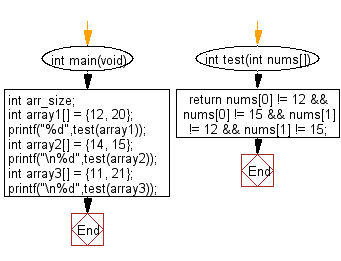﻿ C Program: Arrays of length 2 do not contain 15 or 20# C Exercises: Check whether a given array of integers of length 2, does not contain 15 or 20

## C-programming basic algorithm: Exercise-42 with Solution

Write a C program to check whether a given array of integers of length 2, does not contain 15 or 20.

C Code:

``````#include <stdio.h>
#include <stdlib.h>
int main(void){
int arr_size;
int array1[] = {12, 20};
printf("%d",test(array1));
int array2[] = {14, 15};
printf("\n%d",test(array2));
int array3[] =  {11, 21};
printf("\n%d",test(array3));
}
int test(int nums[])
{
return nums != 12 && nums != 15 && nums != 12 && nums != 15;
}
``````

Sample Output:

```0
0
1
```

Pictorial Presentation:Flowchart:C Programming Code Editor:

What is the difficulty level of this exercise?

Test your Programming skills with w3resource's quiz.

﻿

## C Programming: Tips of the Day

Why doesn't a+++++b work?

printf("%d",a+++++b); is interpreted as (a++)++ + b according to the Maximal Munch Rule!.

++ (postfix) doesn't evaluate to an lvalue but it requires its operand to be an lvalue.

! 6.4/4 says the next preprocessing token is the longest sequence of characters that could constitute a preprocessing token"

Ref : https://bit.ly/3fdldUT